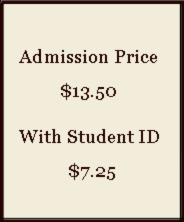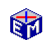Price DiscriminationOffering a student discount is a form of price discrimination that does not entirely displease students.  This EconModel application demonstrates that price discrimination is also profit maximizing behavior for merchants.Model LinkPrice Discrimination
<
Printable PDF Exercises

The application begins by showing you how to graph the demand and profit curves for two groups of customers separately and in the aggregate.  You then determine the profit-maximizing strategy under two scenarios:

• The Base Case -- maximizing profits for a single price
• Price Discrimination -- maximizing profits by charging different prices to different customers

The numerical results confirm the graphical presentation and allow you to calculate the profits to be gained from price discrimination.

Classic Economic Models

Microeconomics

Introduction
Overview of Micro Models

Macroeconomics

Introduction
Overview of Macro Models

Resources